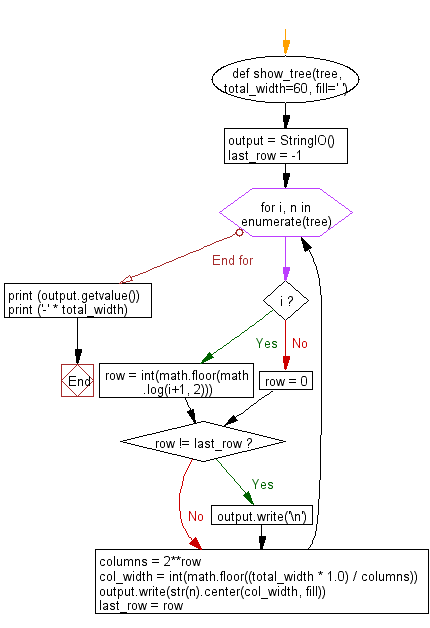﻿ Python heap queue algorithm: Print a heap as a tree-like data structure - w3resource

# Python: Print a heap as a tree-like data structure

## Python heap queue algorithm: Exercise-19 with Solution

Write a Python program to print a heap as a tree-like data structure.

Sample Solution:

Python Code:

``````import math
from io import StringIO
#source https://bit.ly/38HXSoU
def show_tree(tree, total_width=60, fill=' '):
"""Pretty-print a tree.
total_width depends on your input size"""
output = StringIO()
last_row = -1
for i, n in enumerate(tree):
if i:
row = int(math.floor(math.log(i+1, 2)))
else:
row = 0
if row != last_row:
output.write('\n')
columns = 2**row
col_width = int(math.floor((total_width * 1.0) / columns))
output.write(str(n).center(col_width, fill))
last_row = row
print (output.getvalue())
print ('-' * total_width)
return

#test
import heapq
heap = []
heapq.heappush(heap, 1)
heapq.heappush(heap, 2)
heapq.heappush(heap, 3)
heapq.heappush(heap, 4)
heapq.heappush(heap, 7)
heapq.heappush(heap, 9)
heapq.heappush(heap, 10)
heapq.heappush(heap, 8)
heapq.heappush(heap, 16)
heapq.heappush(heap, 14)
show_tree(heap)
```
```

Sample Output:

```                             1
2                             3
4              7              9              10
8      16     14
------------------------------------------------------------
```

Flowchart:Python Code Editor:

Have another way to solve this solution? Contribute your code (and comments) through Disqus.

Next: Write a Python program to combine two given sorted lists using heapq module.

What is the difficulty level of this exercise?

Test your Programming skills with w3resource's quiz.

﻿